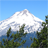# Manual Local Moran's Index calculation

3906
0
02-09-2014 12:25 PMNew Contributor
Dear Forum,

[edit: excel attachment added]

I have been successfully applying the Local Moran's Index (and Gi*) as part of my PhD, but I want to know exactly how the calculation is achieved. I am an archaeologist and I will be writing this in my thesis, so I must present it in a way other non-statisticians can understand it.

I decided to produce a worked example, for Gi* it was simple but I'm having difficulty calculating a single instance of the Local Moran's i (Li).

Thank you in advance for any help you can give me, I really want to crack this as I am just starting my writing up phase (and it's bugging me)!

The ESRI implemented equation is here: http://resources.arcgis.com/en/help/main/10.1/index.html#//005p00000012000000

Here is the local neighbourhood (of a larger dataset 11x11 cells), the target cell is 100.

73|96|82
96|100|92
87|93|82

Neighbourhood average = 87.63

Here is my workings, there is an error which I point out as you go through it.

To get Si^2
sum of al the neighbours minus the mean then squared
this is divided by the number of neighbouring cells minus 1 (the target cell)
this calculation is then subtracted from the mean squared

so:
�??(73-87.63)�??^2+
�??(96-87.63)�??^2+
�??(87-87.63)�??^2+
�??(96-87.63)�??^2+
�??(93-87.63)�??^2+
�??(82-87.63)�??^2+
�??(92-87.63)�??^2+
�??(82-87.63)�??^2     =465.88

then...

Si^2 = 465.88/(8-1)-7678.14= -7611.59

We have to take the value of a neighbour and subtract this from the average of neighbours and multiplied by the search radius (wij)

so:

((73-87.63)*1.75)=-25.60
((96-87.63)*1.75)=14.6475
((87-87.63)*1.75)=-1.1025
((96-87.63)*1.75)=14.6475
((93-87.63)*1.75)=9.3975
((82-87.63)*1.75)=-9.8525
((92-87.63)*1.75)=7.6475
((82-87.63)*1.75)=-9.8525
results summed = -0.07

It should equal -11240.16

This would create the calculation:

I-index=  (100-87.63)/(-7611.)* -11240.16=18.27

Which is what ArcGIS produces, where have I gone wrong?

Thanks, Gary
Tags (2)
0 Replies